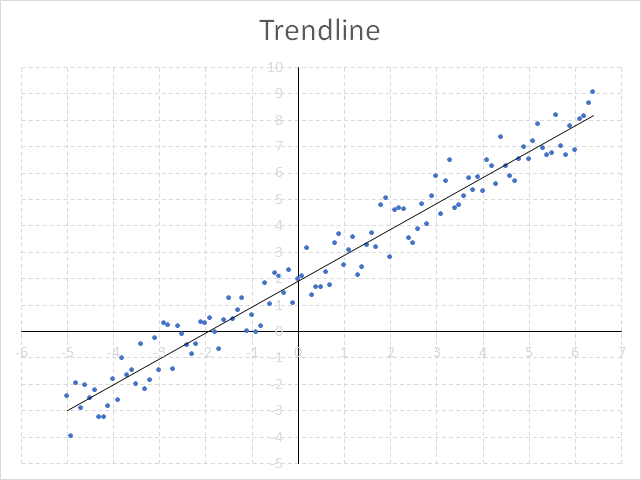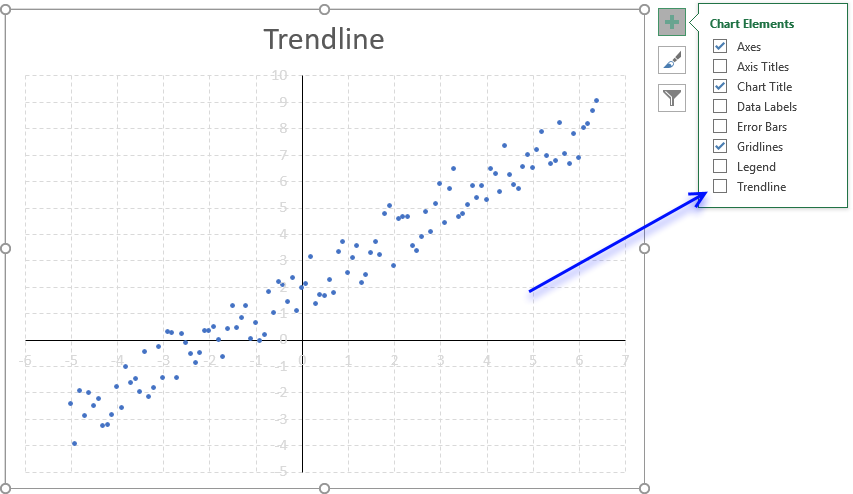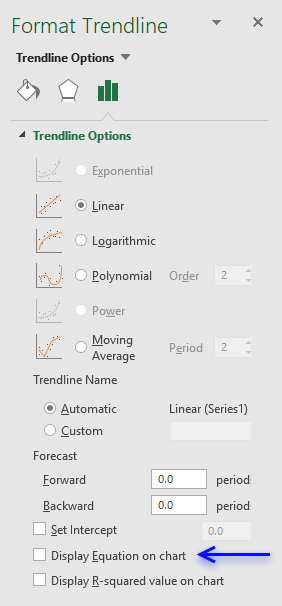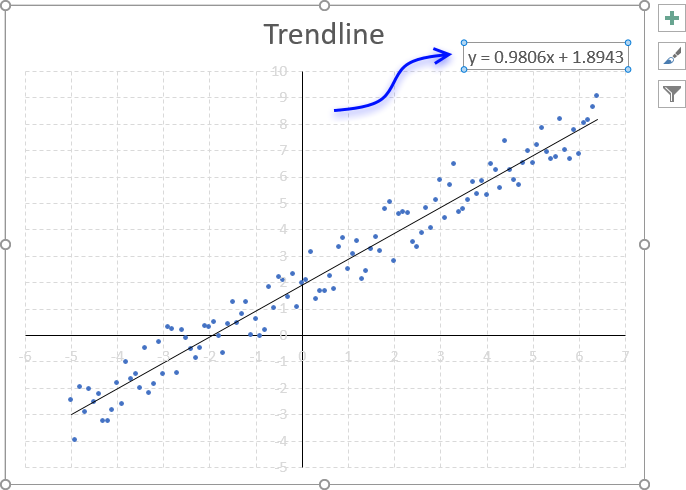Author: Oscar Cronquist Article last updated on October 16, 2018Excel allows you to insert a linear chart trendline that displays a straight line calculated based on the method of least squares of your data series, it is often used in statistical regression analysis.

### How to build

1. Select the chart.
2. Click the "plus" sign next to the chart.
3. Click checkbox "Trendline".### How to display the linear equation on the chart

1. Doubleclick on the trendline to open the settings pane.
2. Click the checkbox "Display Equation on chart".You can build the linear equation manually in Excel using the SLOPE function to get the slope of the regression line. Use the INTERCEPT function to calculate the point at which the line will intersect the y-axis.